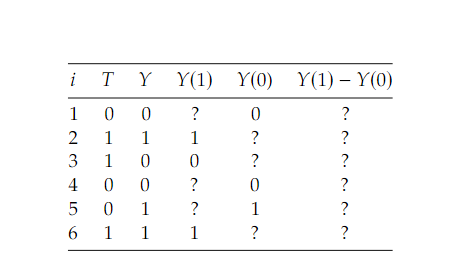Tags
#causality #has-images #statisticsQuestion
Well, what assumption(s) would make it so that the ATE is simply the [...(what type?)] difference? This is equivalent to saying “what makes it valid to calculate the ATE by taking the average of the 𝑌(0) column, ignoring the question marks, and subtracting that from the average of the 𝑌(1) column, ignoring the question marks?” 6 This ignoring of the question marks (missing data) is known as ignorability
associational

Tags
#causality #has-images #statisticsQuestion
Well, what assumption(s) would make it so that the ATE is simply the [...(what type?)] difference? This is equivalent to saying “what makes it valid to calculate the ATE by taking the average of the 𝑌(0) column, ignoring the question marks, and subtracting that from the average of the 𝑌(1) column, ignoring the question marks?” 6 This ignoring of the question marks (missing data) is known as ignorability
?

Tags
#causality #has-images #statisticsQuestion
Well, what assumption(s) would make it so that the ATE is simply the [...(what type?)] difference? This is equivalent to saying “what makes it valid to calculate the ATE by taking the average of the 𝑌(0) column, ignoring the question marks, and subtracting that from the average of the 𝑌(1) column, ignoring the question marks?” 6 This ignoring of the question marks (missing data) is known as ignorability
associational
If you want to change selection, open original toplevel document below and click on "Move attachment"

#### Parent (intermediate) annotation

Open it
Well, what assumption(s) would make it so that the ATE is simply the associational difference? This is equivalent to saying “what makes it valid to calculate the ATE by taking the average of the 𝑌(0) column, ignoring the question marks, and subtracting that from the a

#### Original toplevel document (pdf)

owner: crocodile - (no access) - Introduction_to_Causal_Inference-Nov19_2020-Neal.pdf, p17

#### Summary

status measured difficulty not learned 37% [default] 0

No repetitions